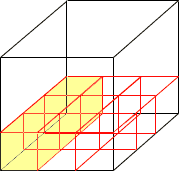How many cubic feet are in a cubic mile? Hi, That's a lot of cubic feet. Let's start with something smaller. How many cubic feet are in a cubic yard? Think about what you would have to do to fill a box 1 yard by 1 yard by 1 yard with boxes that are 1 foot by 1 foot by 1 foot. Since there are 3 feet in a yard you could cover the bottom of the big box with 3 rows of small boxes, each row 3 boxes long. Thus it takes 3 x 3 = 9 cubic foot boxes to cover the bottom of the cubic yard box.Since the cubic yard box is 3 feet high it takes 3 layers to fill it with cubic foot boxes. Thus to fill the cubic yard box you need 3 x 3 x 3 = 27 cubic foot boxes. Hence there are 27 cubic feet in a cubic yard. Now back to your original problem. What if the big box is 1 mile by 1 mile by 1 mile? There are 5280 feet in a mile so to cover the bottom of the box you need 5280 rows of small boxes, each row 5280 boxes long. Thus it takes 5280 x 5280 cubic foot boxes to cover the bottom of the cubic mile box. Since the cubic mile box is 5280 feet high it takes 5280 layers to fill it with cubic foot boxes. Thus to fill the cubic mile box you need 5280 x 5280 x 5280 cubic foot boxes. Penny Go to Math Central